# Hall polynomial

(diff) ← Older revision | Latest revision (diff) | Newer revision → (diff)
Hall polynomials are Lie polynomials obtained from elements of a given Hall set (cf. Lie polynomial; Hall set). They furnish a basis of the free Lie algebra (cf. Lie algebra, free) over a (finite or infinite) set of generators. Elements of a Hall setmay be seen as completely bracketed words (or rooted planar binary trees with leaves labelled by generators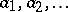; cf. also Binary tree). These are defined recursively as brackets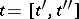, whereare bracketed words of lower weight; bracketed words of weight one correspond to the generators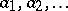. The Hall polynomial associated with the Hall element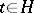is then computed in the free associative ring (i.e. the ring of polynomials with non-commuting indeterminates) following the rule: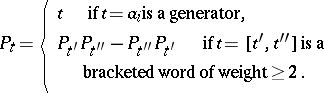A Hall setis totally ordered, thus inducing a total order on the set of polynomials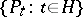. One can show that any non-commutative polynomial is a sum of non-increasing products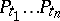of Hall polynomials. This result is the well-known Poincaré–Birkhoff–Witt theorem for free Lie algebras (cf. also Lie algebra, free). One can prove this result combinatorially by first showing that any non-commutative polynomial is a sum of non-increasing products(with non-negative integer coefficients). This is accomplished using rewriting techniques (cf. [a1]); this idea is originally present in [a2]. A theorem stating that any word is a unique non-increasing product of Hall words then implies that these non-increasing products of Hall polynomials form a basis of the free associative algebra. It then follows that the set of Hall polynomials is linearly independent. In order to show that the set of Hall polynomials generate the free Lie algebra, one shows that the bracket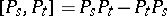of any two Hall polynomials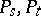is a sum of Hall polynomials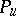with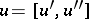and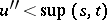. This result is known as the Schützenberger lemma. Consequently, the setis a linear basis for the free Lie algebra over.